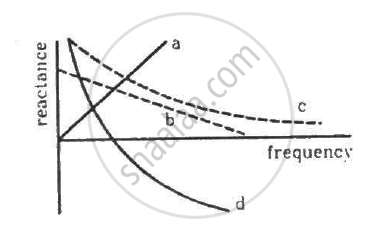Department of Pre-University Education, KarnatakaPUC Karnataka Science Class 12

# Which of the Following Plots May Represent the Reactance of a Series Lc Combination? - Physics

#### Question

Which of the following plots may represent the reactance of a series LC combination?Sum
Short Note

#### Solution

(d)

The reactance of a series LC circuit is given by,
X = X_L - X_c = omegaL - 1/"omegaC"
⇒ X =2pi fL - 1/(2pifC)
The correct relation between the reactance and f is represented by the graph in option (d).
X = 0, "when"  X_L = X_c

Thus plot d represent the reactance of a series LC combination.

Is there an error in this question or solution?

#### APPEARS IN

HC Verma Class 12 Concepts of Physics Vol. 2
Chapter 17 Alternating Current
MCQ | Q 6 | Page 329

#### Video TutorialsVIEW ALL 

Which of the Following Plots May Represent the Reactance of a Series Lc Combination? Concept: Reactance and Impedance.